### Home > CALC > Chapter 1 > Lesson 1.2.3 > Problem1-54

1-54.
1. On graph paper, sketch the function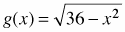. Shade A(g, 3 ≤ x ≤ 6), the region between g(x) and the x-axis, for 3 ≤ x ≤ 6. 1-54 HW eTool (Desmos). Homework Help ✎

1. Use geometry to find this area. Hint: Draw in a radius to create two easier regions whose difference is the shaded region.

2. Find A(g, 0 ≤ x ≤ 3).

3. Find A(g, −3 ≤ x ≤ 6).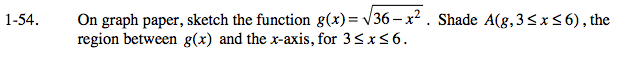You should recognize g as the equation of a semicircle, centered at the origin with radius 6.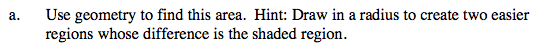View the eTool. The height of the triangle is 5 and the hypotenuse is 6. Use these lengths to calculate the measure of the central angle.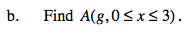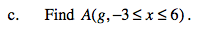Use the eTool below for parts (a) - (c).
Click the link at right for the full version of the eTool: Calc 1-54 HW eTool